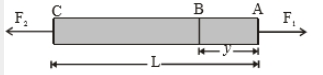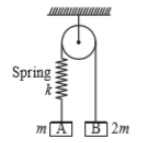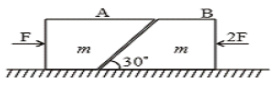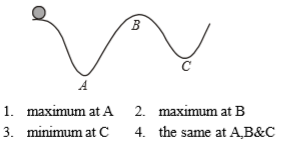A particle is moving in a vertical circle. The tension in the string when passing through two positions at anele of 30$°$ and 60$°$ from vertical (the lowest position) are respectively, then:

1.

2.

3.

4.  Tension in the string always remains the same.

Concept Questions :-

Non-Uniform vertical circular motion
High Yielding Test Series + Question Bank - NEET 2020

Difficulty Level:

A motorcycle is going on an overbridge of radius R. The driver maintains a constant speed. As the motorcycle is ascending on the overbridge, the normal force on it:

1.  Increases

2.  decreases

3.  remains the same

4.  fluctuates erratically

Concept Questions :-

Non-Uniform vertical circular motion
High Yielding Test Series + Question Bank - NEET 2020

Difficulty Level:

A rod of length L and mass m is acted on by two unequal forces ${F}_{1}$ and ${F}_{2}\left(<{F}_{1}\right)$ as shown in the following figureThe tension in the rod at a distance y from the end A is given by :

1. ${F}_{1}\left(1-\frac{y}{L}\right)+{F}_{2}\left(\frac{y}{L}\right)$

2. ${F}_{2}\left(1-\frac{y}{L}\right)+{F}_{1}\left(\frac{y}{L}\right)$

3. $\left({F}_{1}-{F}_{2}\right)\frac{y}{L}$

4. None of the above

Concept Questions :-

Application of laws
High Yielding Test Series + Question Bank - NEET 2020

Difficulty Level:

Two blocks A and B of masses m & 2m respectively are held at rest such that the spring is in natural length. Find the accelerations of both the blocks just after release.(1)

(2)

(3) (0, 0)

(4)

Concept Questions :-

Application of laws
High Yielding Test Series + Question Bank - NEET 2020

Difficulty Level:

Two blocks 'A' and 'B' each of mass 'm' are placed on a smooth horizontal surface. Two horizontal force F and 2F are applied on both the blocks 'A' and 'B' respectively as shown in figure. The block A does not slide on block B. Then the normal reaction acting between the two blocks is:(1) F

(2) F/2

(3) $\frac{F}{\sqrt{3}}$

(4) 3F

Concept Questions :-

Application of laws
High Yielding Test Series + Question Bank - NEET 2020

Difficulty Level:

Five persons A, B, C, D & E are pulling a cart of mass 100 kg on a smooth surface and the cart is moving with acceleration 3 $\mathrm{m}/{\mathrm{s}}^{2}$ in east direction. When person 'A' stops pulling, it moves with acceleration 1 $\mathrm{m}/{\mathrm{s}}^{2}$ in the west direction. When person 'B' stops pulling, it moves with acceleration 24 $\mathrm{m}/{\mathrm{s}}^{2}$ in the north direction. The magnitude of the acceleration of the cart when only A & B pull the cart keeping their directions same as the old directions are:

1.  26 $\mathrm{m}/{\mathrm{s}}^{2}$

2.

3.  25 $\mathrm{m}/{\mathrm{s}}^{2}$

4.  30 $\mathrm{m}/{\mathrm{s}}^{2}$

Concept Questions :-

Application of laws
High Yielding Test Series + Question Bank - NEET 2020

Difficulty Level:

A body moves along an uneven surface with constant speed at all points.

The normal reaction due to ground on the body is :Concept Questions :-

Uniform circular motion
High Yielding Test Series + Question Bank - NEET 2020

Difficulty Level:

The forces required to just move a body up an inclined plane is double the force required to just prevent it from sliding down. If $\mathrm{\varphi }$ is angle of friction and $\mathrm{\theta }$ is the angle which makes with horizontal, then

1.

2.

3.

4.

Concept Questions :-

Friction
High Yielding Test Series + Question Bank - NEET 2020

Difficulty Level:

If no external force acts on the system, then the correct statement is:

1.  velocity of centre of mass changes

2.  momentum of centre of mass is conserved and constant

3.  position of the center of mass changes with time

4.  None of these

Concept Questions :-

Newton laws
High Yielding Test Series + Question Bank - NEET 2020

Difficulty Level:

Two masses m and M are connected by a light string passing over a smooth pulley. When mass m moves up by 1.4 m in 2 sec, the ratio $\frac{m}{M}$ is

(1) $\frac{13}{15}$

(2) $\frac{15}{13}$

(3) $\frac{9}{7}$

(4) $\frac{7}{9}$

Concept Questions :-

Application of laws